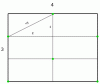BrainDen.com - Brain Teasers
• 0

# Points in a rectangle

## Question

There are 6 points in a rectangle with the sides, 3 and 4. Prove that the distance between at least two of these points is smaller than the square root of 5.

## Recommended Posts

• 0

Square root of five screams out for an hypotenuse, and in this puzzle, that's what it is.

For two points inside a rectangle their greatest separation is the diagonal.

For a 1 x 2 rectangle the diagonal is sqrt(5).

And in a 3 x 4 rectangle, there are exactly six such rectangles.

So a weaker, immediate result applies to seven points: By the pigeon hole principle,

at least two of the seven would be in the same 1 x 2 rectangle and thus be closer than sqrt(5).But the result holds also for six points, as shown in the figure.

Optimally placing six points, all on ends of diagonals, the vertical pairs are only 2 units apart.

##### Share on other sites

• 0

1. I place two points on the diagonal end points.
2. I place two more a distance x from them along the long edges.
3. I place the final two a distance x from them along the short edges.

Requiring nearest points of type 2 and 3 to have a spacing of x gives

x2 - 14x + 25 = 0 or x = 2.10102, somewhat less than 51/2.

Edit:

I suspect there is a configuration where the minimum distance is 51/2.

Edited by bonanova

## Join the conversation

You can post now and register later. If you have an account, sign in now to post with your account.×   Pasted as rich text.   Paste as plain text instead

Only 75 emoji are allowed.

×   Your previous content has been restored.   Clear editor

×   You cannot paste images directly. Upload or insert images from URL.